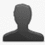new## superscripts only work if there is a space before the phrase

Reported by bohms | June 24th, 2011 @ 10:43 AM

meter^2^ =>

meter^2^

meter ^2^ =>

meter 2

it would be nice to be able to write meter^2^ and get

meter2

a spec fixture to illustrate:
in: meter^2^
html:

meter2

latex: "meter\textsuperscript{2}\n\n"

Thanks for providing such a great library.

### Comments and changes to this ticket

•#### bohmsJune 24th, 2011 @ 10:46 AM

oops, I need to use code blocks here

``` meter^2^ =>```

`meter^2^`

meter ^2^ =>

meter 2

it would be nice to write meter^2^ and get

meter2

A spec fixture

in: meter^2^
150 html:

meter2

151 latex: "meter\textsuperscript{2}\n\n"

Thanks,

•#### bohmsJune 24th, 2011 @ 10:49 AM

Ok one more time, with preview.

``````meter^2^ => <p>meter^2^</p>

meter ^2^ => <p>meter <sup>2</sup></p>

it would be nice to write meter^2^ and get <p>meter<sup>2</sup></p>

A spec fixture:
in: meter^2^
html: <p>meter<sup>2</sup></p>
latex: "meter\\textsuperscript{2}\n\n"```
```

Thanks,

With your very own profile, you can contribute to projects, track your activity, watch tickets, receive and update tickets through your email and much more.